# MICE and KNN missing value imputations Explanation and Implementation with Python

In continuation to my blog on missing values and how to handle them. I am here to talk about 2 more very effective techniques of handling missing data through:

1. MICE or Multiple Imputation by Chained Equation
2. KNN or K-Nearest Neighbor imputation

First we will talk about Multiple Imputation by Chained Equation.

Multiple Imputation by Chained Equation assumes that data is MAR , i.e. missing at random.

Sometimes data missing in a dataset is related to the other features and can be predicted using other feature values.

It cannot be imputed with general ways of using mean, mode, or median.

For example if weight value is missing for a person, he/she may or may not be having diabetes but filling in this value needs evaluation with use of other features like height, BMI, overweight to predict the right set of value.

For doing this linear regression is applied and steps are as below:

Step1:

In data below, we delete few data from the dataset and impute it with mean value using Simple imputer used in univariate imputation.

Now we clearly see a problem here, person with overweight category 2, height 173.8 cm, and BMI 25.7 cannot have weight 67.82 kgs.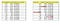Image by Author

Step 2:

Weight value is deleted and rest of the values are kept intact.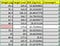Image by Author

Step 3:

Linear regression is then trained on grey cells with Weight as target feature.

White cells is then treated as test data and value is predicted. Suppose value ‘a’ comes for weight.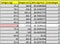Image by Author

Step 4:

We will put ‘a’ value in weight feature and remove value in height feature.

Linear regression is then trained on grey cells with height as target feature.

White cells is then treated as test data and height value is predicted. Suppose value ‘b’ comes for height.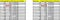Image by Author

Step 5:

We will put ‘b’ value in height feature and remove value in BMI feature next.

Linear regression is then trained on grey cells with BMI as target feature.

White cells is then treated as test data and BMI value is predicted. Suppose value ‘c’ comes for BMI.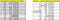Image by Author

Step 6:

Now we subtract base values in step 5 and step 1.

all values comes to 0 except that we imputed which comes as (a-67.82) in weight, (b-165.13) in height and (c-25.81) in BMI.

The target is to minimize these values near to zero in each iteration.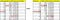Image by Author

For next iteration values of step 5 are kept in step 1 and steps are repeated from 2 to 6.

In Python it is done as:

It is a sophisticated approach is to use the IterativeImputer class, which models each feature with missing values as a function of other features, and uses that estimate for imputation. It is done in an iterated manner and at each step, a feature column is designated as output y and the other feature columns are treated as inputs X. A regressor is fit on (X,y) for known y. Then, the regressor is used to predict the missing values of y. This is done for each feature in an iterative fashion, and then is repeated for max_iter imputation rounds.

`import numpy as npfrom sklearn.experimental import enable_iterative_imputerfrom sklearn.impute import IterativeImputerfrom sklearn.linear_model import LinearRegressionlr = LinearRegression()imp = IterativeImputer(estimator=lr,missing_values=np.nan, max_iter=10, verbose=2, imputation_order='roman',random_state=0)X=imp.fit_transform(X)`

KNN or K-Nearest Neighbor Imputation

K-Nearest Neighbor is one of the simplest and easiest technique of imputation in machine learning. It works on Euclidean distance between the neighbor cordinates X and y to know how similar data is.

This imputation is explained with a very easy example given below: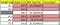Image by Author

Suppose we need to predict weight of row 3 which is missing from the dataset.

All other rows have data and some missing columns as well.

To find out the weights following steps have to be taken:

1) Choose missing value to fill in the data.

2) Select the values in a row

3) Choose the number of neighbors you want to work with (ideally 2–5)

4)Calculate Euclidean distance from all other data points corresponding to each other in the row.

5) Select the smallest 2 and average out.

Calculation of Euclidean distance is :

distance= sqrt(weight*distance from present coordinates)

weight= total number of features to predict a feature divided by number of features having value.

distance of coordinates is calculated as square of following values:

for height=164.7–154.9, 164.7–157.8,164.7–169.9,164.7–154.9

for BMI= 24.7363331759203–29.2156250664228, blank, 24.7363331759203–23.3839037677878,24.7363331759203–28.5071149007606

blank is for BMI value missing in row2.

for Overweight=1–2,1–2,1–1,blank

For above values its is done as under:(Square of values with weights)

For rows 2 and 5 as 1 feature had missing value each weights are 3/2 for rest weights are 3/3.

Distance is calculated as per formula.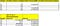Image by Author

So we select 2 nearest values that are 8.5390280477347 and 5.37299404429225 in above case. For these rows weights are 64.9 and 67.5.

So for missing value weight will be average of these 2.

weight= (64.9 + 67.5)/2= 132.4/2= 66.2Kg .

For rest of the missing feature values similar approach is taken.

In Python KNNImputer class provides imputation for filling the missing values using the k-Nearest Neighbors approach. By default, nan_euclidean_distances, is used to find the nearest neighbors ,it is a Euclidean distance metric that supports missing values. Every missing feature is imputed using values from n_neighbors nearest neighbors that have a value of nearest neighbours to be taken into consideration. The feature of the neighbors are averaged uniformly or weighted by distance to each neighbor. If a sample has more than one feature missing, then the neighbors for that sample can be different depending on the particular feature being imputed. There are no defined distances to the training set, the training set used during imputation. If there is at least one neighbor with a defined distance, the weighted or unweighted average of the remaining neighbors will be used during imputation.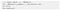Image by Author

Conclusion:

Both MICE and KNN imputations are calculated as per logical reasoning with data and its relation to other features.

It is way above other imputation methods like mean, median, mode, simple imputations or random value imputation. However it is used for MAR category of missing variables.

I hope after reading this blog MICE and KNN imputations must be easy to understand.

Originally published at https://www.numpyninja.com on November 6, 2020.

## Nerd For Tech

From Confusion to Clarification

Written by

## Namrata Kapoor

Data Science Professional | Technical Blogger | Artificial Intelligence | NLP | Chatbots and more

## More From Medium

Medium is an open platform where 170 million readers come to find insightful and dynamic thinking. Here, expert and undiscovered voices alike dive into the heart of any topic and bring new ideas to the surface. Learn more

Follow the writers, publications, and topics that matter to you, and you’ll see them on your homepage and in your inbox. Explore

If you have a story to tell, knowledge to share, or a perspective to offer — welcome home. It’s easy and free to post your thinking on any topic. Write on Medium